## N Adjectives to Describe a Person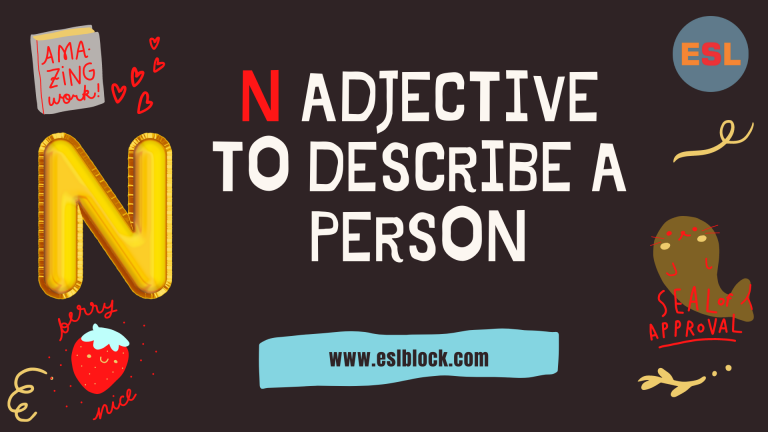There are many different positive adjectives that start with N. And of those, there are many N adjectives to describe a person. Here are some commonly used positive words that start with N, including definitions and examples with a separate…

## M Adjectives to Describe a PersonThere are many different positive adjectives that start with M. And of those, there are many M adjectives to describe a person. Here are some commonly used positive words that start with M, including definitions and examples with a separate…

## L Adjectives to Describe a Person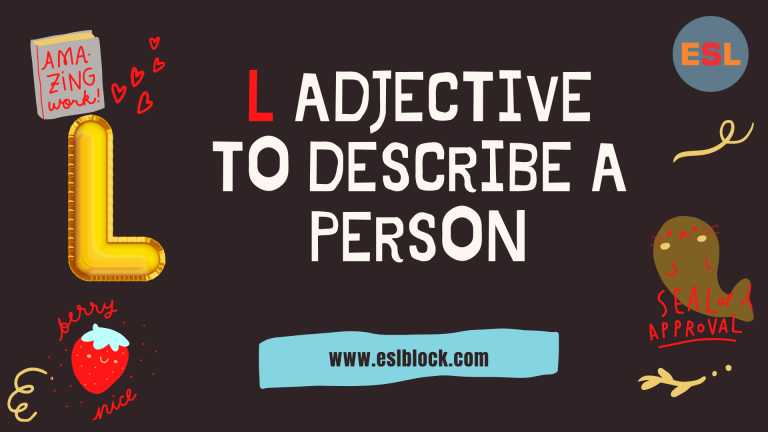There are many different positive adjectives that start with L. And of those, there are many L adjectives to describe a person. Here are some commonly used positive words that start with L, including definitions and examples with a separate…

## K Adjectives to Describe a Person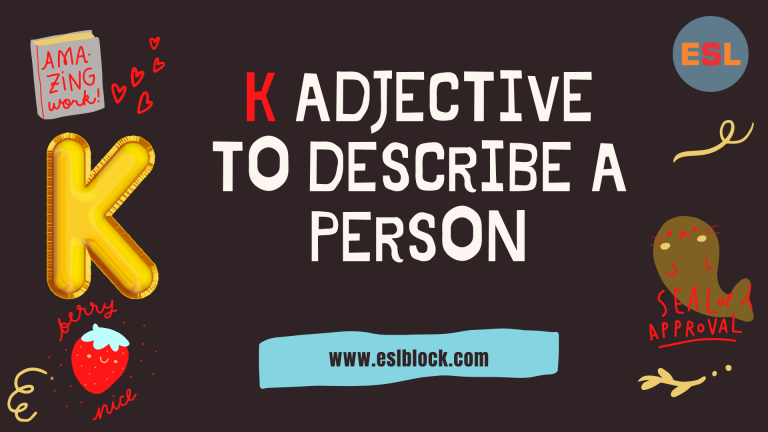There are many different positive adjectives that start with K. And of those, there are many K adjectives to describe a person. Here are some commonly used positive words that start with K, including definitions and examples with a separate…

## J Adjectives to Describe a Person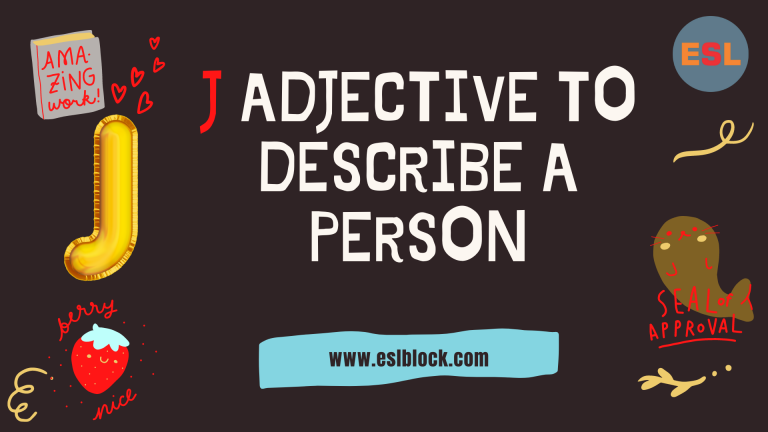There are many different positive adjectives that start with J. And of those, there are many J adjectives to describe a person. Here are some commonly used positive words that start with J, including definitions and examples with a separate…

## I Adjectives to Describe a Person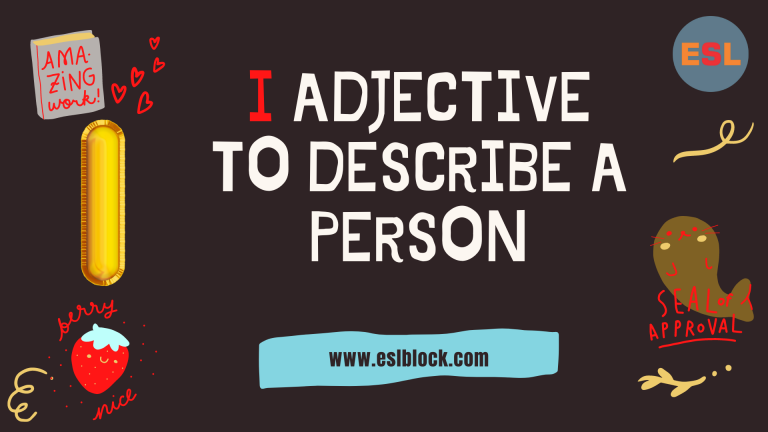There are many different positive adjectives that start with I. And of those, there are many I adjectives to describe a person. Here are some commonly used positive words that start with I, including definitions and examples with a separate…

## H Adjectives to Describe a Person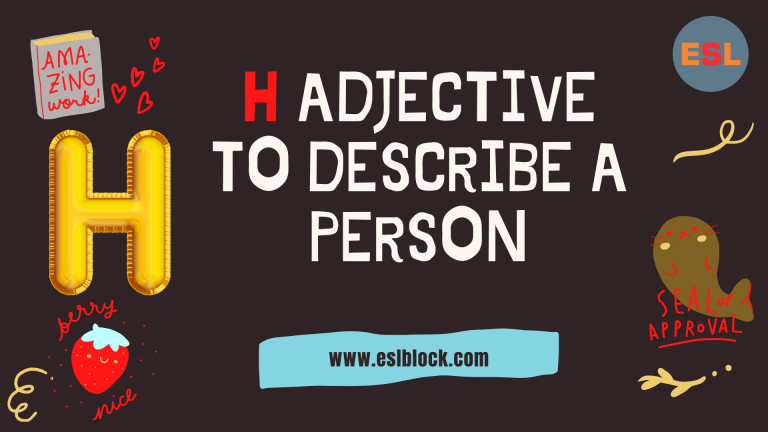There are many different positive adjectives that start with H. And of those, there are many H adjectives to describe a person. Here are some commonly used positive words that start with H, including definitions and examples with a separate…

## G Adjectives to Describe a Person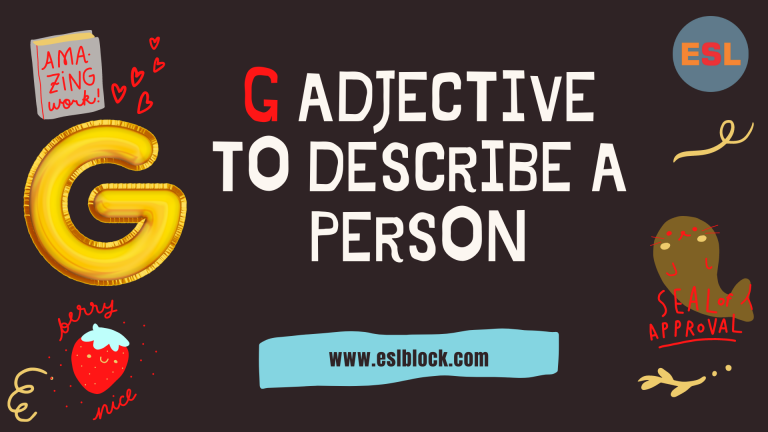There are many different positive adjectives that start with G. And of those, there are many G adjectives to describe a person. Here are some commonly used positive words that start with G, including definitions and examples with a separate…

## F Adjectives to Describe a Person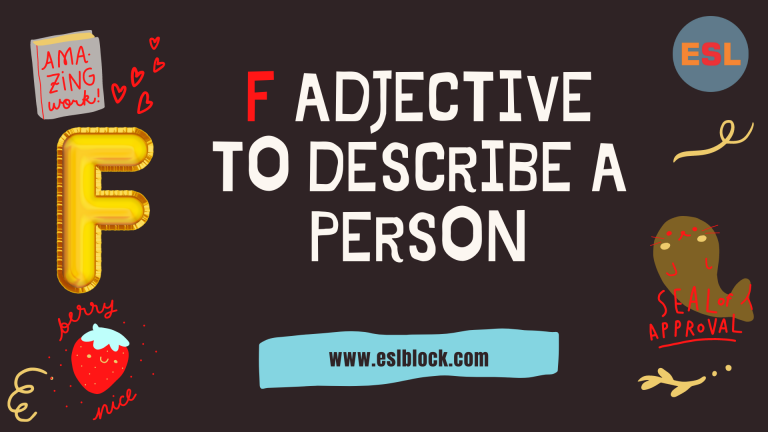There are many different positive adjectives that start with F. And of those, there are many F adjectives to describe a person. Here are some commonly used positive words that start with F, including definitions and examples with a separate…

## E Adjectives to Describe a Person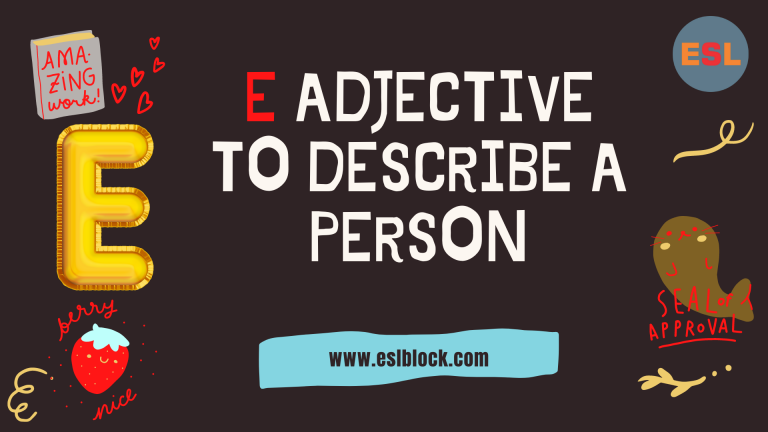There are many different positive adjectives that start with E. And of those, there are many E adjectives to describe a person. Here are some commonly used positive words that start with E, including definitions and examples with a separate…

## D Adjectives to Describe a Person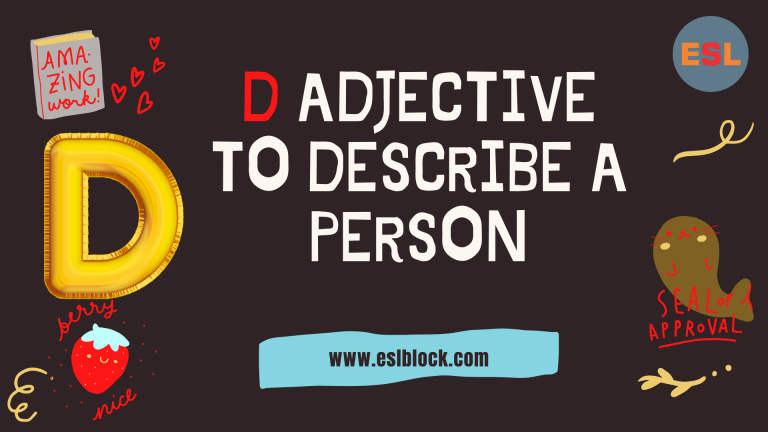There are many different positive adjectives that start with D. And of those, there are many D adjectives to describe a person. Here are some commonly used positive words that start with D, including definitions and examples with a separate…

## C Adjectives to Describe a Person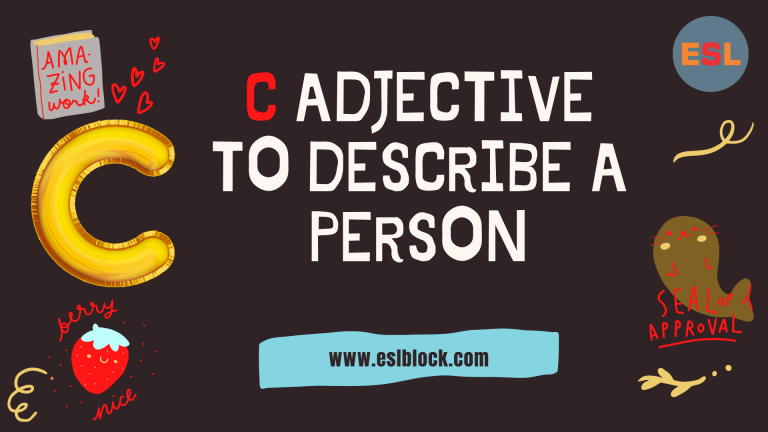There are many different positive adjectives that start with C. And of those, there are many C adjectives to describe a person. Here are some commonly used positive words that start with C, including definitions and examples with a separate…